IIT JAM FollowAkshay Kumar @Eduncle posted an Article
September 23, 2019 • 5:22 pm

# IIT JAM Syllabus 2020 for Physics, Chemistry & Mathematics (Updated)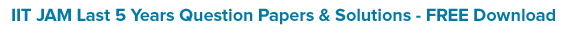Preparations for any entrance exam or be it any nature exam begins smoothly if we have the promptly defined syllabus and the concerned topics of the course subjects.

Admitting yes to the above statement, if any of you engineering Grads who want to pursue M.Sc. or Ph.D. from the premier IITs or IISc, must be looking for precisely and unmistakably defined IIT JAM Syllabus 2020.

You have approached the finest gateway to grab yourself a copy of the prescribed curriculum for entrance exam.

Here at Eduncle.com, you can get complete details for the IIT JAM 2020 syllabus, subject wise. Below you can locate your subject and jot down the relevant JAM Exam contents.

 View IIT JAM Subject Wise Syllabus Download Link IIT JAM Physics Syllabus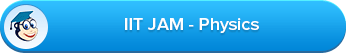IIT JAM Chemistry Syllabus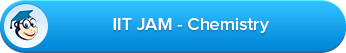IIT JAM Geology Syllabus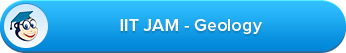IIT JAM Mathematics Syllabus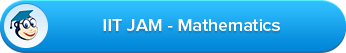IIT JAM Biotechnology Syllabus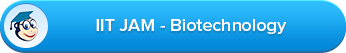IIT JAM Mathematical Statistics Syllabus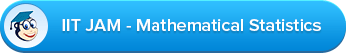IIT JAM BL Syllabus has not been mentioned, as it was discontinued after 2019. Below is the complete prescribed syllabus of IIT JAM all the subjects, You can also download their PDF files through the links given in this blog.

## IIT JAM Physics Syllabus

IIT JAM Physics Syllabus covers all the topics which can be asked in the exam. So, candidates who want to appear in physics must check the complete details of 2020 Syllabus here.

Mathematical Methods: Calculus of single and multiple variables, partial derivatives, Jacobian, imperfect and perfect differentials, Taylor expansion, Fourier series. Vector algebra, Vector Calculus, Multiple integrals, Divergence theorem, Green’s theorem, Stokes’ theorem. First order equations and linear second order differential equations with constant coefficients. Matrices and determinants, Algebra of complex numbers.

Mechanics and General Properties of Matter: Newton’s laws of motion and applications, Velocity and acceleration in Cartesian, polar and cylindrical coordinate systems, uniformly rotating frame, centrifugal and Coriolis forces, Motion under a central force, Kepler’s laws, Gravitational Law and field, Conservative and non-conservative forces. System of particles, Center of mass, equation of motion of the CM, conservation of linear and angular momentum, conservation of energy, variable mass systems. Elastic and inelastic collisions. Rigid body motion, fixed axis rotations, rotation and translation, moments of Inertia and products of Inertia, parallel and perpendicular axes theorem. Principal moments and axes. Kinematics of moving fluids, equation of continuity, Euler’s equation, Bernoulli’s theorem.

Oscillations, Waves and Optics: Differential equation for simple harmonic oscillator and its general solution. Superposition of two or more simple harmonic oscillators. Lissajous figures. Damped and forced oscillators, resonance. Wave equation, traveling and standing waves in one-dimension. Energy density and energy transmission in waves. Group velocity and phase velocity. Sound waves in media. Doppler Effect. Fermat’s Principle. General theory of image formation. Thick lens, thin lens and lens combinations. Interference of light, optical path retardation. Fraunhofer diffraction. Rayleigh criterion and resolving power. Diffraction gratings. Polarization: linear, circular and elliptic polarization. Double refraction and optical rotation.

Electricity and Magnetism: Coulomb’s law, Gauss’s law. Electric field and potential. Electrostatic boundary conditions, Solution of Laplace’s equation for simple cases. Conductors, capacitors, dielectrics, dielectric polarization, volume and surface charges, electrostatic energy. Biot-Savart law, Ampere’s law, Faraday’s law of electromagnetic induction, Self and mutual inductance. Alternating currents. Simple DC and AC circuits with R, L and C components. Displacement current, Maxwell’s equations and plane electromagnetic waves, Poynting’s theorem, reflection and refraction at a dielectric interface, transmission and reflection coefficients (normal incidence only). Lorentz Force and motion of charged particles in electric and magnetic fields.

Kinetic theory, Thermodynamics: Elements of Kinetic theory of gases. Velocity distribution and Equipartition of energy. Specific heat of Mono-, di- and tri-atomic gases. Ideal gas, van der Waals gas and equation of state. Mean free path. Laws of thermodynamics. Zeroth law and concept of thermal equilibrium. First law and its consequences. Isothermal and adiabatic processes. Reversible, irreversible and quasi-static processes. Second law and entropy. Carnot cycle. Maxwell’s thermodynamic relations and simple applications. Thermodynamic potentials and their applications. Phase transitions and Clausius-Clapeyron equation. Ideas of ensembles, Maxwell-Boltzmann, Fermi-Dirac and Bose-Einstein distributions.

Modern Physics: Inertial frames and Galilean invariance. Postulates of special relativity. Lorentz transformations. Length contraction, time dilation. Relativistic velocity addition theorem, mass energy equivalence. Blackbody radiation, photoelectric effect, Compton effect, Bohr’s atomic model, X-rays. Wave-particle duality, Uncertainty principle, the superposition principle, calculation of expectation values, Schrödinger equation and its solution for one, two and three dimensional boxes. Solution of Schrödinger equation for the one dimensional harmonic oscillator. Reflection and transmission at a step potential, Pauli exclusion principle. Structure of atomic nucleus, mass and binding energy. Radioactivity and its applications. Laws of radioactive decay.

Solid State Physics, Devices and Electronics: Crystal structure, Bravais lattices and basis. Miller indices. X-ray diffraction and Bragg's law; Intrinsic and extrinsic semiconductors, variation of resistivity with temperature. Fermi level. p-n junction diode, I-V characteristics, Zener diode and its applications

BJT: characteristics in CB, CE, CC modes. Single stage amplifier, two stage R-C coupled amplifiers.

Simple Oscillators: Barkhausen condition, sinusoidal oscillators. OPAMP and applications: Inverting and non-inverting amplifier.

Boolean algebra: Binary number systems; conversion from one system to another system; binary addition and subtraction. Logic Gates AND, OR, NOT, NAND, NOR exclusive OR; Truth tables; combination of gates; de Morgan's theorem.

## IIT JAM Chemistry Syllabus

In Chemistry, IIT JAM Syllabus 2020 is divided into three parts - Physical Chemistry, Organic Chemistry and Inorganic chemistry. Below, we have mentioned the section wise syllabus for chemistry. You can download the PDF here and start your preparation now.

IIT JAM Syllabus 2020 - PHYSICAL CHEMISTRY

Basic Mathematical Concepts: Functions; maxima and minima; integrals; ordinary differential equations; vectors and matrices; determinants; elementary statistics and probability theory.

Atomic and Molecular Structure: Fundamental particles; Bohr’s theory of hydrogen-like atom; wave-particle duality; uncertainty principle; Schrödinger’s wave equation; quantum numbers; shapes of orbitals; Hund’s rule and Pauli’s exclusion principle; electronic configuration of simple homonuclear diatomic molecules.

Theory of Gasses: Equation of state for ideal and non-ideal (van der Waals) gases; Kinetic theory of gases; Maxwell-Boltzmann distribution law; equipartition of energy.

Solid state: Crystals and crystal systems; X-rays; NaCl and KCl structures; close packing; atomic and ionic radii; radius ratio rules; lattice energy; Born-Haber cycle; isomorphism; heat capacity of solids.

Chemical Thermodynamics: Reversible and irreversible processes; first law and its application to ideal and non-ideal gases; thermochemistry; second law; entropy and free energy; criteria for spontaneity.

Chemical and Phase Equilibria: Law of mass action; Kp, Kc, Kx and Kn; effect of temperature on K; ionic equilibria in solutions; pH and buffer solutions; hydrolysis; solubility product; phase equilibria–phase rule and its application to one-component and two-component systems; colligative properties.

Electrochemistry: Conductance and its applications; transport number; galvanic cells; EMF and free energy; concentration cells with and without transport; polarography; concentration cells with and without transport; Debey-Huckel-Onsagar theory of strong electrolytes.

Chemical Kinetics: Reactions of various order; Arrhenius equation; collision theory; transition state theory; chain reactions - normal and branched; enzyme kinetics; photochemical processes; catalysis.

Spectroscopy: Beer-Lambert law; fundamental concepts of rotational, vibrational, electronic and magnetic resonance spectroscopy.

IIT JAM Syllabus 2020 - ORGANIC CHEMISTRY

Basic Concepts in Organic Chemistry and Stereochemistry: Electronic effects (resonance, inductive, hyperconjugation) and steric effects and its applications (acid/base property); optical isomerism in compounds with and without any stereocenters (allenes, biphenyls); conformation of acyclic systems (substituted ethane/n-propane/n-butane) and cyclic systems (mono- and di-substituted cyclohexanes).

Organic Reaction Mechanism and Synthetic Applications: Chemistry of reactive intermediates (carbocations, carbanions, free radicals, carbenes, nitrenes, benzynes etc…); Hofmann-Curtius-Lossen rearrangement, Wolff rearrangement, Simmons-Smith reaction, Reimer-Tiemann reaction, Michael reaction, Darzens reaction, Wittig reaction and McMurry reaction; Pinacol-pinacolone, Favorskii, benzilic acid rearrangement, dienone-phenol rearrangement, Baeyer-Villeger reaction; oxidation and reduction reactions in organic chemistry; organometallic reagents in organic synthesis (Grignard, organolithium and organocopper); Diels-Alder, electrocyclic and sigmatropic reactions; functional group inter-conversions and structural problems using chemical reactions.

Qualitative Organic Analysis: Identification of functional groups by chemical tests; elementary UV, IR and 1H NMR spectroscopic techniques as tools for structural elucidation.

Natural Products Chemistry: Chemistry of alkaloids, steroids, terpenes, carbohydrates, amino acids, peptides and nucleic acids.

Aromatic and Heterocyclic Chemistry: Monocyclic, bicyclic and tricyclic aromatic hydrocarbons, and monocyclic compounds with one hetero atom: synthesis, reactivity and properties.

IIT JAM Syllabus 2020 - INORGANIC CHEMISTRY

Periodic Table: Periodic classification of elements and periodicity in properties; general methods of isolation and purification of elements.

Chemical Bonding and Shapes of Compounds: Types of bonding; VSEPR theory and shapes of molecules; hybridization; dipole moment; ionic solids; structure of NaCl, CsCl, diamond and graphite; lattice energy.

Main Group Elements (s and p blocks): General concepts on group relationships and gradation in properties; structure of electron deficient compounds involving main group elements.

Transition Metals (d block): Characteristics of 3d elements; oxide, hydroxide and salts of first row metals; coordination complexes: structure, isomerism, reaction mechanism and electronic spectra; VB, MO and Crystal Field theoretical approaches for structure, color and magnetic properties of metal complexes; organometallic compounds having ligands with back bonding capabilities such as metal carbonyls, carbenes, nitrosyls and metallocenes; homogenous catalysis.

Bioinorganic Chemistry: Essentials and trace elements of life; basic reactions in the biological systems and the role of metal ions, especially Fe2+, Fe3+, Cu2+ and Zn2+; structure and function of hemoglobin and myoglobin and carbonic anhydrase.

Instrumental Methods of Analysis: Basic principles; instrumentations and simple applications of conductometry, potentiometry and UV-vis spectrophotometry; analysis of water, air and soil samples.

Analytical Chemistry: Principles of qualitative and quantitative analysis; acid-base, oxidation-reduction and complexometric titrations using EDTA; precipitation reactions; use of indicators; use of organic reagents in inorganic analysis; radioactivity; nuclear reactions; applications of isotopes.

## IIT JAM Mathematics Syllabus

Before starting your 2020 Preparation, one question arises in your mind - How to crack Joint Admission Test? And, the answer is if you cover the complete IIT JAM Syllabus 2020 with best study plan then you can crack it.  The mathematics include the following topics which are: -

Sequences and Series of Real Numbers: Sequence of real numbers, convergence of sequences, bounded and monotone sequences, convergence criteria for sequences of real numbers, Cauchy sequences, subsequences, Bolzano-Weierstrass theorem. Series of real numbers, absolute convergence, tests of convergence for series of positive terms – comparison test, ratio test, root test; Leibniz test for convergence of alternating series.

Functions of One Real Variable: Limit, continuity, intermediate value property, differentiation, Rolle’s Theorem, Mean Value Theorem, L'Hospital rule, Taylor's theorem, Maxima and Minima.

Functions of Two or Three Real Variables: Limit, continuity, partial derivatives, differentiability, maxima and minima.

Integral Calculus: Integration as the inverse process of differentiation, definite integrals and their properties, fundamental theorem of calculus. Double and triple integrals, change of order of integration, calculating surface areas and volumes using double integrals, calculating volumes using triple integrals.

Differential Equations: Ordinary differential equations of the first order of the form y'=f(x,y), Bernoulli’s equation, exact differential equations, integrating factor, orthogonal trajectories, homogeneous differential equations, variable separable equations,  linear differential equations of second order with constant coefficients, method of variation of parameters, Cauchy-Euler equation.

Vector Calculus: Scalar and vector fields, gradient, divergence, curl, line integrals, surface integrals, Green, Stokes and Gauss theorems.

Group Theory: Groups, subgroups, Abelian groups, non-Abelian groups, cyclic groups, permutation groups, normal subgroups, Lagrange's Theorem for finite groups, group homomorphisms and basic concepts of quotient groups.

Linear Algebra: Finite dimensional vector spaces, linear independence of vectors, basis, dimension, linear transformations, matrix representation, range space, null space, rank-nullity theorem. Rank and inverse of a matrix, determinant, solutions of systems of linear equations, consistency conditions, eigen values and eigenvectors for matrices, Cayley-Hamilton theorem.

Real Analysis: Interior points, limit points, open sets, closed sets, bounded sets, connected sets, compact sets, completeness of R. Power series (of real variable), Taylor’s series, radius and interval of convergence, term-wise differentiation and integration of power series.

## IIT JAM Mathematical Statistics Syllabus

There is no chance to get the seat in IITs, IISc for MSc withoutthis exam. If you don’t know the IIT JAM Statistics Syllabus 2020 then how can you crack it? The Mathematical Statistics (MS) test paper comprises of Mathematics (40% weightage) and Statistics (60% weightage). Below, you can check the Syllabus for Mathematical statistics.

JAM 2020 Syllabus - MATHEMATICS

Sequences and Series: Convergence of sequences of real numbers, Comparison, root and ratio tests for convergence of series of real numbers.

Differential Calculus: Limits, continuity and differentiability of functions of one and two variables. Rolle's theorem, mean value theorems, Taylor's theorem, indeterminate forms, maxima and minima of functions of one and two variables.

Integral Calculus: Fundamental theorems of integral calculus. Double and triple integrals, applications of definite integrals, arc lengths, areas and volumes.

Matrices: Rank, inverse of a matrix. Systems of linear equations. Linear transformations, eigenvalues and eigenvectors. Cayley-Hamilton theorem, symmetric, skew-symmetric and orthogonal matrices.

JAM 2020 Syllabus - STATISTICS

Probability: Axiomatic definition of probability and properties, conditional probability, multiplication rule. Theorem of total probability. Bayes' theorem and independence of events.

Random Variables: Probability mass function, probability density function, and cumulative distribution functions, distribution of a function of a random variable. Mathematical expectation, moments and moment generating function. Chebyshev's inequality.

Standard Distributions: Binomial, negative binomial, geometric, Poisson, hypergeometric, uniform, exponential, gamma, beta and normal distributions. Poisson and normal approximations of a binomial distribution.

Joint Distributions: Joint, marginal and conditional distributions. Distribution of functions of random variables. Joint moment generating function. Product moments, correlation, simple linear regression. Independence of random variables.

Sampling distributions: Chi-square, t and F distributions, and their properties.

Limit Theorems: Weak law of large numbers. Central limit theorem (i.i.d. with finite variance case only).

Estimation: Unbiasedness, consistency and efficiency of estimators, method of moments and method of maximum likelihood. Sufficiency, factorization theorem. Completeness, Rao-Blackwell and Lehmann- Scheffe theorems, uniformly minimum variance unbiased estimators. Rao-Cramer inequality. Confidence intervals for the parameters of univariate normal, two independent normals, and one parameter exponential distributions.

Testing of Hypotheses: Basic concepts, applications of Neyman-Pearson Lemma for testing simple and composite hypotheses. Likelihood ratio tests for parameters of the univariate normal distribution.

## IIT JAM Biotechnology Syllabus

The IIT JAM Biotechnology Syllabus 2020 comprises of Biology (44% weightage), Chemistry (20% weightage), Mathematics (18% weightage) and Physics (18% weightage).

IIT JAM Syllabus 2020 - BIOLOGY (10+2+3 level)

General Biology: Taxonomy; Heredity; Genetic variation; Conservation; Principles of ecology; Evolution; Techniques in modern biology.

Biochemistry and Physiology: Carbohydrates; Proteins; Lipids; Nucleic acids; Enzymes; Vitamins; Hormones; Metabolism - Glycolysis, TCA cycle, Oxidative Phosphoryation; Photosynthesis. Nitrogen Fixation, Fertilization and Osmoregulation; Vertebrates-Nervous system; Endocrine system; Vascular system; Immune system; Digestive system and Reproductive System.

Basic Biotechnology: Tissue culture; Application of enzymes; Antigen-antibody interaction; Antibody production; Diagnostic aids.

Molecular Biology: DNA; RNA; Replication; Transcription; Translation; Proteins; Lipids and Membranes; Operon model; Gene transfer.

Cell Biology: Cell cycle; Cytoskeletal elements; Mitochondria; Endoplasmic reticulum; Chloroplast; Golgi apparatus; Signaling.

Microbiology: Isolation; Cultivation; Structural features of virus; Bacteria; Fungi; Protozoa; Pathogenic micro-organisms.

IIT JAM 2020 Syllabus - CHEMISTRY (10+2+3 level)

Atomic Structure: Bohr’s theory and Schrodinger wave equation; Periodicity in properties; Chemical bonding; Properties of s, p, d and f block elements; Complex formation; Coordination compounds; Chemical equilibria; Chemical thermodynamics (first and second law); Chemical kinetics (zero, first, second and third order reactions); Photochemistry; Electrochemistry; Acid-base concepts; Stereochemistry of carbon compounds; Inductive, electromeric, conjugative effects and resonance; Chemistry of Functional Groups: Hydrocarbons, alkyl halides, alcohols, aldehydes, ketones, carboxylic acids, amines and their derivatives; Aromatic hydrocarbons, halides, nitro and amino compounds, phenols, diazonium salts, carboxylic and sulphonic acids; Mechanism of organic reactions; Soaps and detergents; Synthetic polymers; Biomolecules – amino acids, proteins, nucleic acids, lipids and carbohydrates (polysaccharides); Instrumental techniques – chromatography (TLC, HPLC), electrophoresis, UV-Vis, IR and NMR spectroscopy, mass spectrometry.

IIT JAM Syllabus 2020 - MATHEMATICS (10+2 level)

Sets, Relations and Functions, Mathematical Induction, Logarithms, Complex numbers, Linear and Quadratic equations, Sequences and Series, Trigonometry, Cartesian System of Rectangular Coordinates, Straight lines and Family, Circles, Conic Sections, Permutations and Combinations, Binomial Theorem, Exponential and Logarithmic Series, Mathematical Logic, Statistics, Three Dimensional Geometry, Vectors, Matrices and Determinants, Boolean Algebra, Probability, Functions, limits and Continuity, Differentiation, Application of Derivatives, Definite and Indefinite Integrals, Differential Equations.

IIT JAM 2020 Syllabus - PHYSICS (10+2 level)

Physical World and Measurement, Elementary Statics and Dynamics, Kinematics, Laws of Motion, Work, Energy and Power, Electrostatics, Current electricity, Magnetic Effects of Current and Magnetism, Electromagnetic Induction and Alternating Current, Electromagnetic waves, Optics, Dual Nature of Matter and Radiations, Atomic Nucleus, Solids and Semiconductor Devices, Principles of Communication, Motion of System of Particles and Rigid Body, Gravitation, Mechanics of Solids and Fluids, Heat and Thermodynamics, Oscillations, Waves.

## IIT JAM Geology Syllabus

As per the previous analysis, Geology has the highest cut off marks in the exam. Candidates who want to qualify for this exam must cover the complete IIT JAM Geology Syllabus 2020 so that they can easily solve all the questions and get selected in the exam.

The Planet Earth: Origin of the Solar System and the Earth; Geosphere and the composition of the Earth; Shape and size of the earth; Earth-moon system; Formation of continents and oceans; Dating rocks and age of the Earth; Volcanism and volcanic landforms; Interior of earth; Earthquakes; Earth's magnetism and gravity, Isostasy; Elements of Plate tectonics; Orogenic cycles.

Geomorphology: Weathering and erosion; Transportation and deposition due to the wind, ice, river, sea, and resulting landforms, Structurally controlled landforms.

Structural Geology: Concept of stratum; Contour; Outcrop patterns; Maps and cross sections; Dip and strike; Classification and origin of folds, faults, joints, unconformities, foliations and lineations; shear zones. Stereographic and equal area projections of planes and lines; computation of true thickness of beds from outcrops and bore-holes.

Palaeontology: Major steps in the evolution of life forms; Fossils; their mode of preservation and utility; Morphological characters, major evolutionary trends and ages of important groups of animals - Brachiopoda, Mollusca, Trilobita, Graptolitoidea, Anthozoa, Echinodermata; Gondwana plant fossils; Elementary idea of verterbrate fossils in India.

Stratigraphy: Principles of stratigraphy; Litho-, chrono- and biostratigraphic classification; distribution and classification of the stratigraphic horizons of India from Archaean to Recent.

Mineralogy: Symmetry and forms in common crystal classes; Physical properties of minerals; Isomorphism and polymorphism, Classification of minerals; Structure of silicates; Mineralogy of common rock-forming minerals; Mode of occurrence of minerals in rocks. Transmitted polarised light microscopy and optical properties of uniaxial and biaxial minerals.

Petrology: Definition and classification of rocks; Igneous rocks-forms of igneous bodies; Crystallization from magma; classification, association and genesis of igneous rocks; Sedimentary rocks - classification, texture and structure; size and shape of sedimentary bodies. Metamorphic rocks - classification, facies, zones and texture. Characteristic mineral assemblages of pelites in the Barrovian zones and mafic rocks in common facies.

Economic Geology: Properties of common economic minerals; General processes of formation of mineral deposits; Physical characters; Mode of occurrence and distribution in India both of metallic and non-metallic mineral deposits; Coal and petroleum occurrences in India.

Applied Geology: Ground Water; Principles of Engineering Geology.

## What should be the approach to crack IIT JAM Exam 2020?

Cracking the Joint Admission Exam in the very first attempt is the main goal of many B.Sc. Graduates who want to pursue M.Sc from the premier institution like IITs and IISc. It requires a lot of hard work & dedication to crack Joint Admission Test Exam. But, if you have the right approach & strategy to prepare for the exam, then believe us, you can crack the exam in the first attempt easily.

On our website, we will give you medicine for your headache & hiccups for 2020. We, here, will give you vital preparation tips for 2020 Exam and guide you to what to do for the exam, what to prepare for the exam? How to approach the exam? How to increase your chances to get selected in it?

## What Eduncle.com Provides for Joint Admission Test 2020?

Here, you can find the detailed study material & guidance for Joint Admision Test & various such exams. Our faculty & experts have several years of experience in their related field. Eduncle.com also guides the candidates as to how they can improve their performance.

The study material we provide is well researched by our experts and covers IIT JAM Exam Syllabus comprehensively. The innovative approach adopted by us makes the student more confident at the time of examination.

The study material provided by Eduncle for Joint Admission Exam have the following features:

1. Topics are covered as per prescribed Syllabus.

2. Deep extensive coverage of theory to build up a solid foundation and clear concepts.

3. Every phase develops concepts through illustrations and exercises.

4. Mock Test Paper included.

5. Still have confusion? Ask our experts! 1800-120-1021 (Toll Free).

Q.1) Does the IIT JAM syllabus change every year?

No, IIT JAM Syllabus does not change every year by IIT. But, it can be similar to the BSc course. There are seven subjects in it and every subject has a different syllabus.

Q.2) Is there one book that covers the whole syllabus of IIT JAM?

There are no books that covers the complete syllabus of IIT JAM. You need 2-3 books or a preparation course to cover all the topics. If you want to get the Syllabus in a single notebook or document, then you should buy IIT JAM Study Material.

Q.3) What is the syllabus for IIT JAM MSc in Microbiology?

None of the IITs provides Microbiology Syllabus. Microbiology is a part of Biology and Biotechnology. You can download IIT JAM Microbiology Syllabus here.

Q.4) Does JAM entrance is strictly based on the given syllabus?

Yes, JAM Exam is completely based on the given syllabus. So, if you are preparing for JAM then you should cover the whole syllabus of your subject.

Q.5) How many topics are there in IIT JAM Physics syllabus. What are the minimum number of topics needed to score well in it? Which are they?

There are 10 topics in IIT JAM Physics Syllabus which are: -

Mathematical Methods

Mechanics and General Properties of Matter

Oscillations, Waves and Optics

Electricity and Magnetism

Kinetic theory, Thermodynamics

Modern Physics

Solid State Physics, Devices and Electronics

BJT

Simple Oscillators

Boolean algebra

If you cover the below topics, then you can get more than 50 marks in the Physics Exam.

Mechanics and General Properties of Matter

Solid State Physics, Devices and Electronics

Electricity and Magnetism

Oscillations, Waves, and Optics

Essential Information here

JAM Online Application Instructions [Must Read]

We have covered every single detail regarding the IIT JAM Syllabus in the blog. If you have any queries regarding the same, you can ask us in the comment section below. We'll be happy to assist you!

Happy Preparing Folks!

You Might Also Like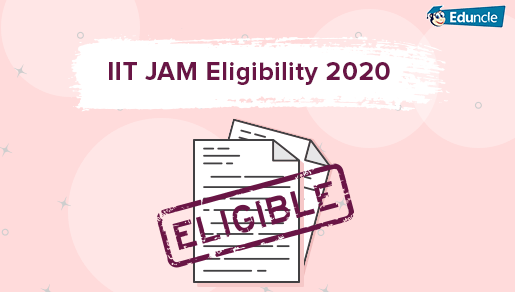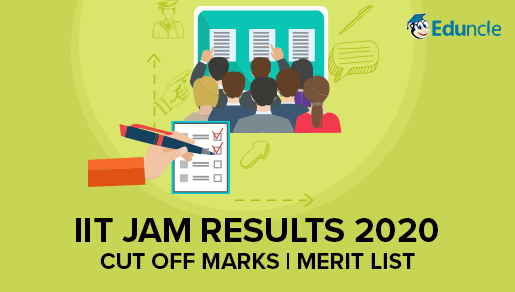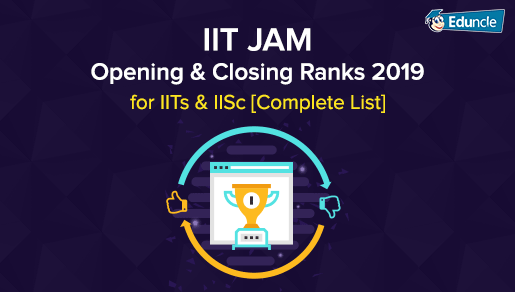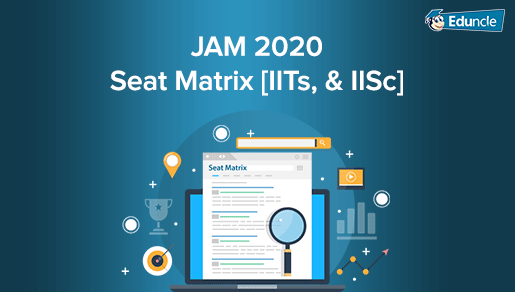Showing 21 comments out of 28
• HARISH SAHU
sir kounsa book achha rhta hai iit jam ki exam ki tayari ke liye??
Eduncle Team @Eduncle
Hello Harish,   Kindly mention your subject for JAM preparation and I'll suggest you the most recommended books for that.   Thanks for asking!   Kind Regards, Eduncl...
Eduncle Team @Eduncle
Hello Harish,   Refer the link - IIT JAM Books.   Thanks for aski...
• Geetika Jain
I am not able to download the syllabus for iit jam chemistry
Eduncle Team @Eduncle
Hello Geetika,   Greetings from Eduncle!   Thanks for asking your query with us!   Call us at our toll-free no - 1800-120-1021. Our expert will guide you.   Kin...
• Bidisha Dey
Sir, please suggest me some books for biological science for prepation of iit jam
Eduncle Team @Eduncle
Hello Bidisha Dey,   You can check out the IIT JAM Biological Science Books b...
• Chirag Verma
Eduncle Team @Eduncle
• Vishal Sharma
sir i want the notes for the preparation for iit jam from mathematics
Eduncle Team @Eduncle
• Anisha
Up to what rank are students selected for IIT's college
Eduncle Team @Eduncle
Hello Anisha,   Greetings from Eduncle!   Upto 1000 candidates get IITs or IISC as college. Remaining can apply for NITs.   Hope the above information will be helpfu...
• Sabari Giri
I can't be able to download notes regarding differential and integral calculus.
Eduncle Team @Eduncle
Hello Sabri,   May we know which subject are you preparing for?
• Dhruba
To registration in iit jam i have to complete my graduation?
Eduncle Team @Eduncle
Hello Dhruba,   Yes! To appear in IIT JAM, you must have graduation degree. To know more visit -
• Nibedita sahu
sir plz suggest me how i go to iit jam now i am student of +3 physics.......
Eduncle Team @Eduncle
Hello Nibedita, Greetings from Eduncle! First, you should fill the IIT JAM application form and start your preparation for JAM Physics. Thanks for asking your query with us! Kind R...
• Deepti
Sir I am not able to download previous year question paper of geology
Eduncle Team @Eduncle
Hello Deepti,
Greeting From Eduncle!
•